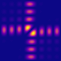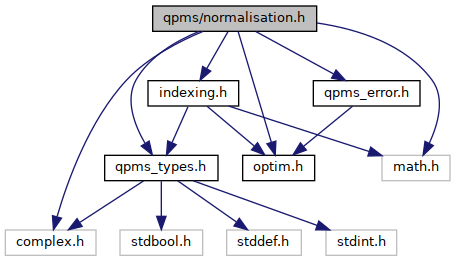QPMS Electromagnetic multiple scattering library and toolkit.
normalisation.h File Reference

Convention-dependent coefficients for VSWFs. More...

#include "qpms_types.h"
#include "qpms_error.h"
#include <math.h>
#include <complex.h>
#include "indexing.h"
#include "optim.h"
Include dependency graph for normalisation.h:Go to the source code of this file.

## Functions

static double qpms_normalisation_normfactor (qpms_normalisation_t norm, qpms_l_t l, qpms_m_t m)
Returns the (real positive) common norm factor of a given normalisation compared to the reference convention. More...

static complex double qpms_normalisation_factor_M_noCS (qpms_normalisation_t norm, qpms_l_t l, qpms_m_t m)
Returns the factors of a magnetic basis VSWF of a given convention compared to the reference convention. More...

static complex double qpms_normalisation_factor_M (qpms_normalisation_t norm, qpms_l_t l, qpms_m_t m)
Returns the factors of a magnetic basis VSWF of a given convention compared to the reference convention. More...

static complex double qpms_normalisation_factor_N_noCS (qpms_normalisation_t norm, qpms_l_t l, qpms_m_t m)
Returns the factors of a electric basis VSWF of a given convention compared to the reference convention. More...

static complex double qpms_normalisation_factor_N (qpms_normalisation_t norm, qpms_l_t l, qpms_m_t m)
Returns the factors of a electric basis VSWF of a given convention compared to the reference convention. More...

static complex double qpms_normalisation_factor_N_M (qpms_normalisation_t norm, qpms_l_t l, qpms_m_t m)
Returns the factors of a electric basis VSWF divided by the factor of a magnetic VWFS of a given convention, compared to the reference one.

static complex double qpms_normalisation_factor_L_noCS (qpms_normalisation_t norm, qpms_l_t l, qpms_m_t m)
Returns the factors of a longitudinal basis VSWF of a given convention compared to the reference convention. More...

static complex double qpms_normalisation_factor_L (qpms_normalisation_t norm, qpms_l_t l, qpms_m_t m)
Returns the factors of a longitudinal basis VSWF of a given convention compared to the reference convention. More...

static complex double qpms_normalisation_factor_uvswfi (const qpms_normalisation_t norm, qpms_uvswfi_t ui)
Returns the factors of a basis VSWF of a given convention compared to the reference convention.

static qpms_normalisation_t qpms_normalisation_dual (qpms_normalisation_t norm)
Returns normalisation flags corresponding to the dual spherical harmonics / waves. More...

static complex double qpms_spharm_azimuthal_part (qpms_normalisation_t norm, qpms_m_t m, double phi)
Returns the asimuthal part of a spherical harmonic. More...

static complex double qpms_spharm_azimuthal_part_derivative_div_m (qpms_normalisation_t norm, qpms_m_t m, double phi)
Returns derivative of the asimuthal part of a spherical harmonic divided by m. More...

## Detailed Description

Convention-dependent coefficients for VSWFs.

## ◆ qpms_normalisation_dual()

 static qpms_normalisation_t qpms_normalisation_dual ( qpms_normalisation_t norm )
inlinestatic

Returns normalisation flags corresponding to the dual spherical harmonics / waves.

This reverses the normalisation factors returned by qpms_normalisation_factor_* and conjugates the asimuthal part for complex spherical harmonics, $$e^{\pm im\phi} \leftrightarrow e^{\mp im\phi}$$.

## ◆ qpms_normalisation_factor_L()

 static complex double qpms_normalisation_factor_L ( qpms_normalisation_t norm, qpms_l_t l, qpms_m_t m )
inlinestatic

Returns the factors of a longitudinal basis VSWF of a given convention compared to the reference convention.

This version takes into account the Condon-Shortley phase bit. Do not use if the C.-S. has already been taken into account e.g. in a gsl_sf_legendre_*_e() call.

## ◆ qpms_normalisation_factor_L_noCS()

 static complex double qpms_normalisation_factor_L_noCS ( qpms_normalisation_t norm, qpms_l_t l, qpms_m_t m )
inlinestatic

Returns the factors of a longitudinal basis VSWF of a given convention compared to the reference convention.

This version ignores the Condon-Shortley phase bit (perhaps because the Condon-Shortley phase is already taken into account in a gsl_sf_legendre_*_e() call.)

## ◆ qpms_normalisation_factor_M()

 static complex double qpms_normalisation_factor_M ( qpms_normalisation_t norm, qpms_l_t l, qpms_m_t m )
inlinestatic

Returns the factors of a magnetic basis VSWF of a given convention compared to the reference convention.

This version takes into account the Condon-Shortley phase bit. Do not use if the C.-S. has already been taken into account e.g. in a gsl_sf_legendre_*_e() call.

## ◆ qpms_normalisation_factor_M_noCS()

 static complex double qpms_normalisation_factor_M_noCS ( qpms_normalisation_t norm, qpms_l_t l, qpms_m_t m )
inlinestatic

Returns the factors of a magnetic basis VSWF of a given convention compared to the reference convention.

This version ignores the Condon-Shortley phase bit (perhaps because the Condon-Shortley phase is already taken into account in a gsl_sf_legendre_*_e() call.)

## ◆ qpms_normalisation_factor_N()

 static complex double qpms_normalisation_factor_N ( qpms_normalisation_t norm, qpms_l_t l, qpms_m_t m )
inlinestatic

Returns the factors of a electric basis VSWF of a given convention compared to the reference convention.

This version takes into account the Condon-Shortley phase bit. Do not use if the C.-S. has already been taken into account e.g. in a gsl_sf_legendre_*_e() call.

## ◆ qpms_normalisation_factor_N_noCS()

 static complex double qpms_normalisation_factor_N_noCS ( qpms_normalisation_t norm, qpms_l_t l, qpms_m_t m )
inlinestatic

Returns the factors of a electric basis VSWF of a given convention compared to the reference convention.

This version ignores the Condon-Shortley phase bit (perhaps because the Condon-Shortley phase is already taken into account in a gsl_sf_legendre_*_e() call.)

## ◆ qpms_normalisation_normfactor()

 static double qpms_normalisation_normfactor ( qpms_normalisation_t norm, qpms_l_t l, qpms_m_t m )
inlinestatic

Returns the (real positive) common norm factor of a given normalisation compared to the reference convention.

Does NOT perform the inversion if QPMS_NORMALISATION_INVERSE is set.

## ◆ qpms_spharm_azimuthal_part()

 static complex double qpms_spharm_azimuthal_part ( qpms_normalisation_t norm, qpms_m_t m, double phi )
inlinestatic

Returns the asimuthal part of a spherical harmonic.

Returns

$e^{im\phi}$

for standard complex spherical harmonics,

$e^{-im\phi$

for complex spherical harmonics and QPMS_NORMALISATION_REVERSE_AZIMUTHAL_PHASE set.

For real spherical harmonics, this gives

$\sqrt{2}\cos{m \phi} \quad \mbox{if } m>0, \\ \sqrt{2}\sin{m \phi} \quad \mbox{if } m<0, \\ 0 \quad \mbox{if } m>0. \\$

## ◆ qpms_spharm_azimuthal_part_derivative_div_m()

 static complex double qpms_spharm_azimuthal_part_derivative_div_m ( qpms_normalisation_t norm, qpms_m_t m, double phi )
inlinestatic

Returns derivative of the asimuthal part of a spherical harmonic divided by m.

This is used to evaluate the VSWFs together with the pi member array of the qpms_pitau_t structure.

Returns

$i e^{im\phi}$

for standard complex spherical harmonics,

$-i e^{-i\phi$

for complex spherical harmonics and QPMS_NORMALISATION_REVERSE_AZIMUTHAL_PHASE set.

For real spherical harmonics, this gives

$-\sqrt{2}\sin{m \phi} \quad \mbox{if } m>0, \\ \sqrt{2}\cos{m \phi} \quad \mbox{if } m<0, \\ -1 \quad \mbox{if } \mbox{if }m=0. \\$

(The value returned for $$m = 0$$ should not actually be used for anything except for multiplying by zero.)